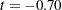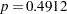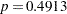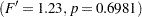## Example 95.1 Using Summary Statistics to Compare Group Means

This example, taken from Huntsberger and Billingsley (1989), compares two grazing methods using 32 steers. Half of the steers are allowed to graze continuously while the other half are subjected to controlled grazing time. The researchers want to know if these two grazing methods affect weight gain differently. The data are read by the following DATA step:

```data graze;
length GrazeType \$ 10;
input GrazeType \$ WtGain @@;
datalines;
controlled  45   controlled  62
controlled  96   controlled 128
controlled 120   controlled  99
controlled  28   controlled  50
controlled 109   controlled 115
controlled  39   controlled  96
controlled  87   controlled 100
controlled  76   controlled  80
continuous  94   continuous  12
continuous  26   continuous  89
continuous  88   continuous  96
continuous  85   continuous 130
continuous  75   continuous  54
continuous 112   continuous  69
continuous 104   continuous  95
continuous  53   continuous  21
;
run;
```

The variable GrazeType denotes the grazing method: "controlled" is controlled grazing and "continuous" is continuous grazing. The dollar sign (\$) following GrazeType makes it a character variable, and the trailing at signs (@@) tell the procedure that there is more than one observation per line.

If you have summary data—that is, just means and standard deviations, as computed by PROC MEANS—then you can still use PROC TTEST to perform a simpletest analysis. This example demonstrates this mode of input for PROC TTEST. Note, however, that graphics are unavailable when summary statistics are used as input.

The MEANS procedure is invoked to create a data set of summary statistics with the following statements:

```proc sort;
by GrazeType;
proc means data=graze noprint;
var WtGain;
by GrazeType;
output out=newgraze;
run;
```

The NOPRINT option eliminates all printed output from the MEANS procedure. The VAR statement tells PROC MEANS to compute summary statistics for the WtGain variable, and the BY statement requests a separate set of summary statistics for each level of GrazeType. The OUTPUT OUT= statement tells PROC MEANS to put the summary statistics into a data set called newgraze so that it can be used in subsequent procedures. This new data set is displayed in Output 95.1.1 by using PROC PRINT as follows:

```proc print data=newgraze;
run;
```

The _STAT_ variable contains the names of the statistics, and the GrazeType variable indicates which group the statistic is from.

Output 95.1.1 Output Data Set of Summary Statistics
Obs GrazeType _TYPE_ _FREQ_ _STAT_ WtGain
1 continuous 0 16 N 16.000
2 continuous 0 16 MIN 12.000
3 continuous 0 16 MAX 130.000
4 continuous 0 16 MEAN 75.188
5 continuous 0 16 STD 33.812
6 controlled 0 16 N 16.000
7 controlled 0 16 MIN 28.000
8 controlled 0 16 MAX 128.000
9 controlled 0 16 MEAN 83.125
10 controlled 0 16 STD 30.535

The following statements invoke PROC TTEST with the newgraze data set, as denoted by the DATA= option:

```proc ttest data=newgraze;
class GrazeType;
var WtGain;
run;
```

The CLASS statement contains the variable that distinguishes between the groups being compared, in this case GrazeType. The summary statistics and confidence intervals are displayed first, as shown in Output 95.1.2.

Output 95.1.2 Summary Statistics and Confidence Limits
The TTEST Procedure

Variable: WtGain

GrazeType N Mean Std Dev Std Err Minimum Maximum
continuous 16 75.1875 33.8117 8.4529 12.0000 130.0
controlled 16 83.1250 30.5350 7.6337 28.0000 128.0
Diff (1-2)   -7.9375 32.2150 11.3897

GrazeType Method Mean 95% CL Mean Std Dev 95% CL Std Dev
continuous   75.1875 57.1705 93.2045 33.8117 24.9768 52.3300
controlled   83.1250 66.8541 99.3959 30.5350 22.5563 47.2587
Diff (1-2) Pooled -7.9375 -31.1984 15.3234 32.2150 25.7434 43.0609
Diff (1-2) Satterthwaite -7.9375 -31.2085 15.3335

In Output 95.1.2, The GrazeType column specifies the group for which the statistics are computed. For each class, the sample size, mean, standard deviation and standard error, and maximum and minimum values are displayed. The confidence bounds for the mean are also displayed; however, since summary statistics are used as input, the confidence bounds for the standard deviation of the groups are not calculated.

Output 95.1.3 shows the results of tests for equal group means and equal variances.

Output 95.1.3 t Tests
Method Variances DF t Value Pr > |t|
Pooled Equal 30 -0.70 0.4912
Satterthwaite Unequal 29.694 -0.70 0.4913

Equality of Variances
Method Num DF Den DF F Value Pr > F
Folded F 15 15 1.23 0.6981

A group test statistic for the equality of means is reported for both equal and unequal variances. Both tests indicate a lack of evidence for a significant difference between grazing methods (andfor the pooled test,andfor the Satterthwaite test). The equality of variances test does not indicate a significant difference in the two variances. Note that this test assumes that the observations in both data sets are normally distributed; this assumption can be checked in PROC UNIVARIATE by using the NORMAL option with the raw data.

Although the ability to use summary statistics as input is useful if you lack access to the original data, some of the output that would otherwise be produced in an analysis on the original data is unavailable. There are also limitations on the designs and distributional assumptions that can be used with summary statistics as input. For more information, see the section Input Data Set of Statistics.